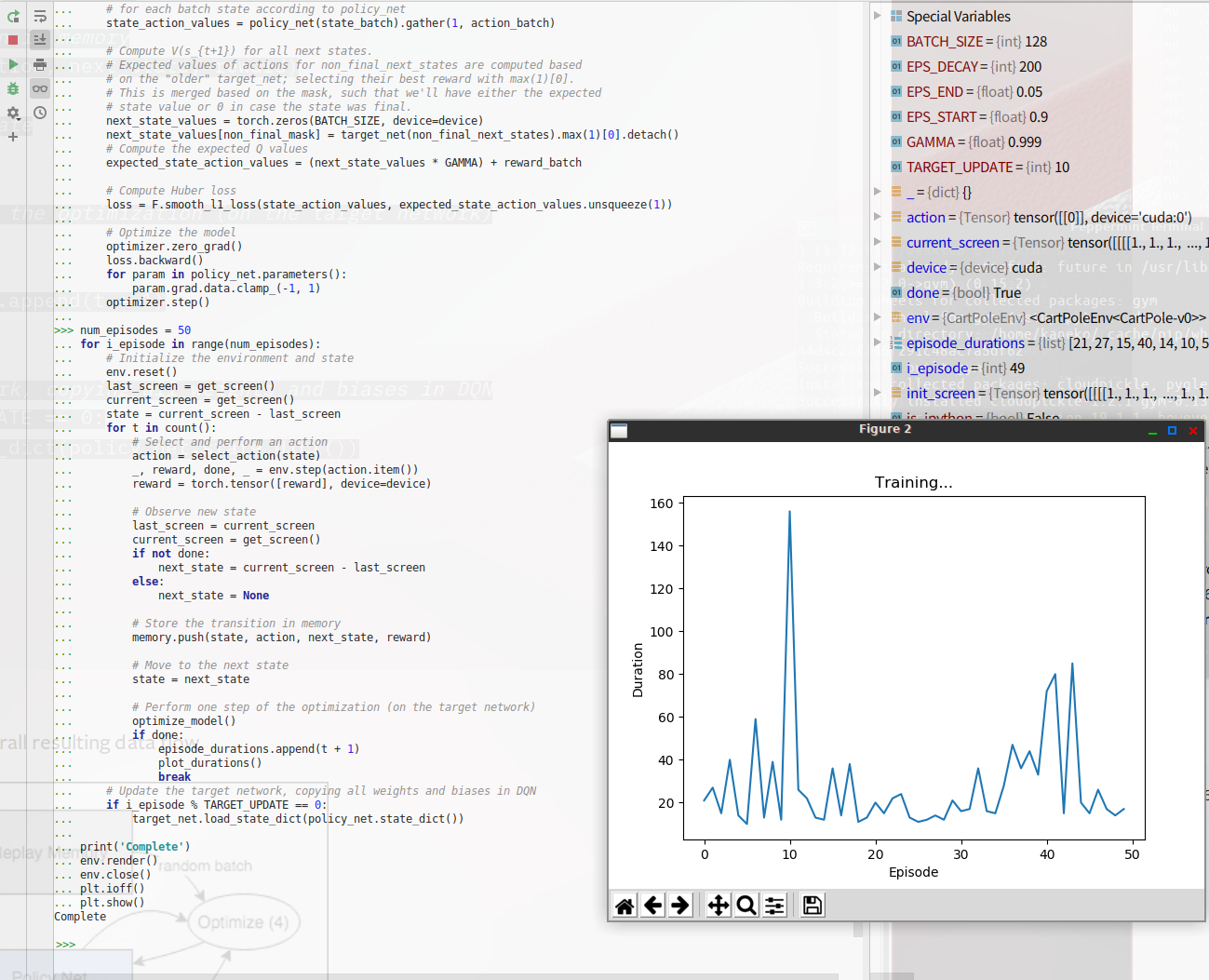金子邦彦研究室人工知能PyTorch の応用例深層強化学習 CartPole-v0 を動かしてみる（PyTorch のサンプルプログラムを使用）

# 深層強化学習 CartPole-v0 を動かしてみる（PyTorch のサンプルプログラムを使用）

ユースケース: 深層強化学習 CartPole-v0 を動かしてみたい

PyTorch の Web ページ: http://pytorch.org

GitHub の PyTorch の Webページ: https://github.com/pytorch/pytorch

## 前準備

### Open-AI gym のインストール

• Ubuntu の場合

次のコマンドを実行．

```sudo pip3 install gym
```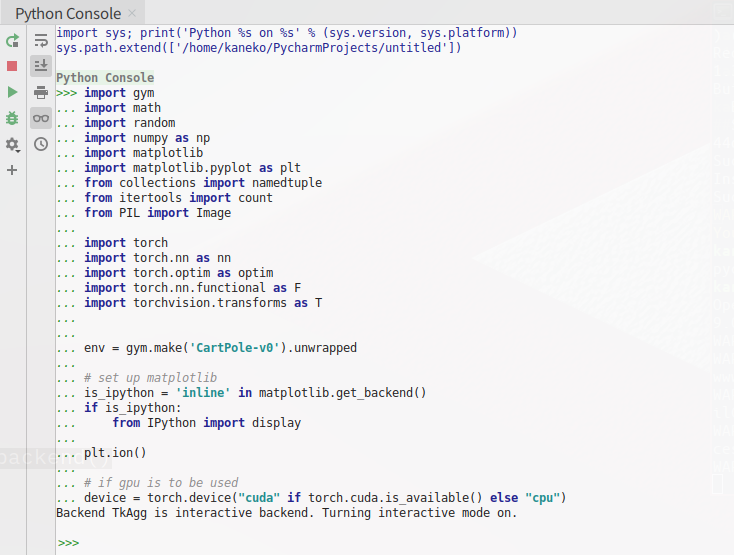## 深層強化学習 CartPole-v0 を動かしてみる（PyTorch のサンプルプログラムを使用）

Python プログラムを実行する

Python プログラムの実行: で説明

Python のまとめ: にまとめ

1. インポート

```import gym
import math
import random
import numpy as np
import matplotlib
%matplotlib inline
import matplotlib.pyplot as plt
import warnings
warnings.filterwarnings('ignore')   # Suppress Matplotlib warnings
from collections import namedtuple
from itertools import count
from PIL import Image

import torch
import torch.nn as nn
import torch.optim as optim
import torch.nn.functional as F
import torchvision.transforms as T

env = gym.make('CartPole-v0').unwrapped

# set up matplotlib
is_ipython = 'inline' in matplotlib.get_backend()
if is_ipython:
from IPython import display

plt.ion()

# if gpu is to be used
device = torch.device("cuda:0" if torch.cuda.is_available() else "cpu")
```

Ubuntu での実行結果例2. CIFAR 10 のダウンロード

```transform = transforms.Compose(
[transforms.ToTensor(),
transforms.Normalize((0.5, 0.5, 0.5), (0.5, 0.5, 0.5))])

classes = ('plane', 'car', 'bird', 'cat', 'deer', 'dog', 'frog', 'horse', 'ship', 'truck')
```

Ubuntu の PyCharm での実行結果例3. Replay Memory の作成

```Transition = namedtuple('Transition',
('state', 'action', 'next_state', 'reward'))

class ReplayMemory(object):

def __init__(self, capacity):
self.capacity = capacity
self.memory = []
self.position = 0

def push(self, *args):
"""Saves a transition."""
if len(self.memory) < self.capacity:
self.memory.append(None)
self.memory[self.position] = Transition(*args)
self.position = (self.position + 1) % self.capacity

def sample(self, batch_size):
return random.sample(self.memory, batch_size)

def __len__(self):
return len(self.memory)
```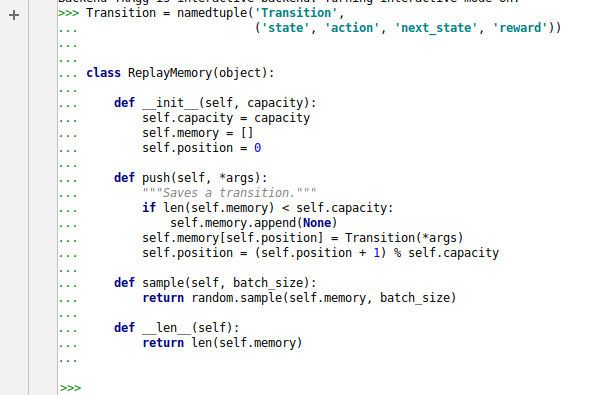4. Q-network の作成

```class DQN(nn.Module):

def __init__(self, h, w, outputs):
super(DQN, self).__init__()
self.conv1 = nn.Conv2d(3, 16, kernel_size=5, stride=2)
self.bn1 = nn.BatchNorm2d(16)
self.conv2 = nn.Conv2d(16, 32, kernel_size=5, stride=2)
self.bn2 = nn.BatchNorm2d(32)
self.conv3 = nn.Conv2d(32, 32, kernel_size=5, stride=2)
self.bn3 = nn.BatchNorm2d(32)

# Number of Linear input connections depends on output of conv2d layers
# and therefore the input image size, so compute it.
def conv2d_size_out(size, kernel_size = 5, stride = 2):
return (size - (kernel_size - 1) - 1) // stride  + 1
convw = conv2d_size_out(conv2d_size_out(conv2d_size_out(w)))
convh = conv2d_size_out(conv2d_size_out(conv2d_size_out(h)))
linear_input_size = convw * convh * 32

# Called with either one element to determine next action, or a batch
# during optimization. Returns tensor([[left0exp,right0exp]...]).
def forward(self, x):
x = F.relu(self.bn1(self.conv1(x)))
x = F.relu(self.bn2(self.conv2(x)))
x = F.relu(self.bn3(self.conv3(x)))
```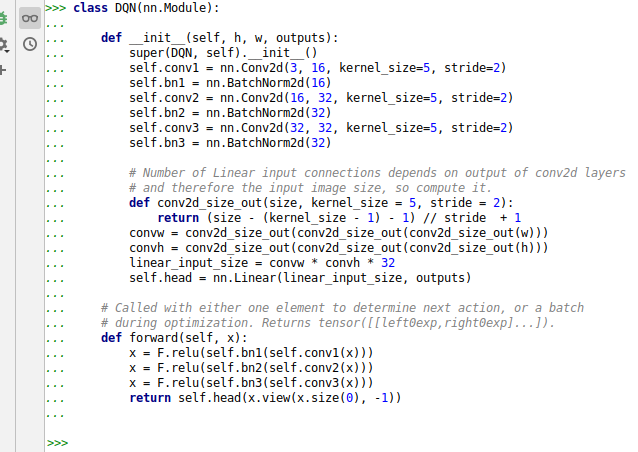5. Input Extraction (入力の抽出)

```resize = T.Compose([T.ToPILImage(),
T.Resize(40, interpolation=Image.CUBIC),
T.ToTensor()])

def get_cart_location(screen_width):
world_width = env.x_threshold * 2
scale = screen_width / world_width
return int(env.state * scale + screen_width / 2.0)  # MIDDLE OF CART

def get_screen():
# Returned screen requested by gym is 400x600x3, but is sometimes larger
# such as 800x1200x3. Transpose it into torch order (CHW).
screen = env.render(mode='rgb_array').transpose((2, 0, 1))
# Cart is in the lower half, so strip off the top and bottom of the screen
_, screen_height, screen_width = screen.shape
screen = screen[:, int(screen_height*0.4):int(screen_height * 0.8)]
view_width = int(screen_width * 0.6)
cart_location = get_cart_location(screen_width)
if cart_location < view_width // 2:
slice_range = slice(view_width)
elif cart_location > (screen_width - view_width // 2):
slice_range = slice(-view_width, None)
else:
slice_range = slice(cart_location - view_width // 2,
cart_location + view_width // 2)
# Strip off the edges, so that we have a square image centered on a cart
screen = screen[:, :, slice_range]
# Convert to float, rescale, convert to torch tensor
# (this doesn't require a copy)
screen = np.ascontiguousarray(screen, dtype=np.float32) / 255
screen = torch.from_numpy(screen)
# Resize, and add a batch dimension (BCHW)
return resize(screen).unsqueeze(0).to(device)

env.reset()
plt.figure()
plt.imshow(get_screen().cpu().squeeze(0).permute(1, 2, 0).numpy(),
interpolation='none')
plt.title('Example extracted screen')
plt.show()
```6. ハイパーパラメータなど

```BATCH_SIZE = 128
GAMMA = 0.999
EPS_START = 0.9
EPS_END = 0.05
EPS_DECAY = 200
TARGET_UPDATE = 10

# Get screen size so that we can initialize layers correctly based on shape
# returned from AI gym. Typical dimensions at this point are close to 3x40x90
# which is the result of a clamped and down-scaled render buffer in get_screen()
init_screen = get_screen()
_, _, screen_height, screen_width = init_screen.shape

# Get number of actions from gym action space
n_actions = env.action_space.n

policy_net = DQN(screen_height, screen_width, n_actions).to(device)
target_net = DQN(screen_height, screen_width, n_actions).to(device)
target_net.eval()

optimizer = optim.RMSprop(policy_net.parameters())
memory = ReplayMemory(10000)

steps_done = 0

def select_action(state):
global steps_done
sample = random.random()
eps_threshold = EPS_END + (EPS_START - EPS_END) * \
math.exp(-1. * steps_done / EPS_DECAY)
steps_done += 1
if sample > eps_threshold:
# t.max(1) will return largest column value of each row.
# second column on max result is index of where max element was
# found, so we pick action with the larger expected reward.
return policy_net(state).max(1).view(1, 1)
else:

episode_durations = []

def plot_durations():
plt.figure(2)
plt.clf()
durations_t = torch.tensor(episode_durations, dtype=torch.float)
plt.title('Training...')
plt.xlabel('Episode')
plt.ylabel('Duration')
plt.plot(durations_t.numpy())
# Take 100 episode averages and plot them too
if len(durations_t) >= 100:
means = durations_t.unfold(0, 100, 1).mean(1).view(-1)
means = torch.cat((torch.zeros(99), means))
plt.plot(means.numpy())

plt.pause(0.001)  # pause a bit so that plots are updated
if is_ipython:
display.clear_output(wait=True)
display.display(plt.gcf())
```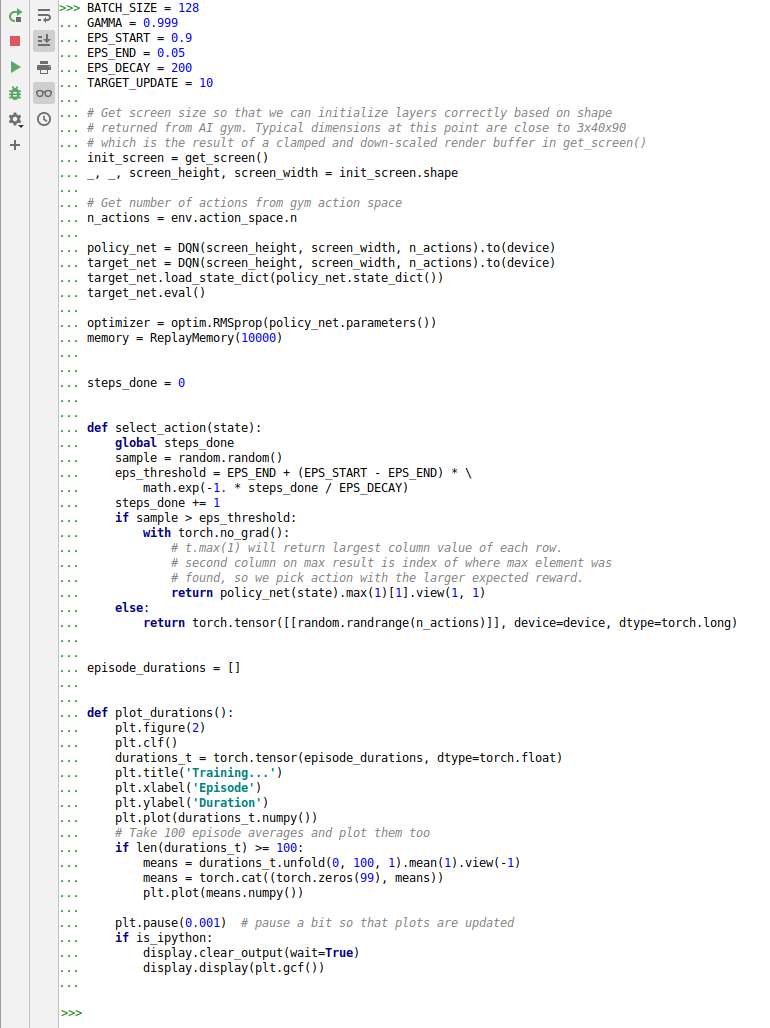7. 最適化の1ステップを実行する関数

```def optimize_model():
if len(memory) < BATCH_SIZE:
return
transitions = memory.sample(BATCH_SIZE)
# Transpose the batch (see https://stackoverflow.com/a/19343/3343043 for
# detailed explanation). This converts batch-array of Transitions
# to Transition of batch-arrays.
batch = Transition(*zip(*transitions))

# Compute a mask of non-final states and concatenate the batch elements
# (a final state would've been the one after which simulation ended)
non_final_mask = torch.tensor(tuple(map(lambda s: s is not None,
batch.next_state)), device=device, dtype=torch.uint8)
non_final_next_states = torch.cat([s for s in batch.next_state
if s is not None])
state_batch = torch.cat(batch.state)
action_batch = torch.cat(batch.action)
reward_batch = torch.cat(batch.reward)

# Compute Q(s_t, a) - the model computes Q(s_t), then we select the
# columns of actions taken. These are the actions which would've been taken
# for each batch state according to policy_net
state_action_values = policy_net(state_batch).gather(1, action_batch)

# Compute V(s_{t+1}) for all next states.
# Expected values of actions for non_final_next_states are computed based
# on the "older" target_net; selecting their best reward with max(1).
# This is merged based on the mask, such that we'll have either the expected
# state value or 0 in case the state was final.
next_state_values = torch.zeros(BATCH_SIZE, device=device)
# Compute the expected Q values
expected_state_action_values = (next_state_values * GAMMA) + reward_batch

# Compute Huber loss
loss = F.smooth_l1_loss(state_action_values, expected_state_action_values.unsqueeze(1))

# Optimize the model
loss.backward()
for param in policy_net.parameters():
optimizer.step()
```8. 学習

```num_episodes = 50
for i_episode in range(num_episodes):
# Initialize the environment and state
env.reset()
last_screen = get_screen()
current_screen = get_screen()
state = current_screen - last_screen
for t in count():
# Select and perform an action
action = select_action(state)
_, reward, done, _ = env.step(action.item())
reward = torch.tensor([reward], device=device)

# Observe new state
last_screen = current_screen
current_screen = get_screen()
if not done:
next_state = current_screen - last_screen
else:
next_state = None

# Store the transition in memory
memory.push(state, action, next_state, reward)

# Move to the next state
state = next_state

# Perform one step of the optimization (on the target network)
optimize_model()
if done:
episode_durations.append(t + 1)
plot_durations()
break
# Update the target network, copying all weights and biases in DQN
if i_episode % TARGET_UPDATE == 0: